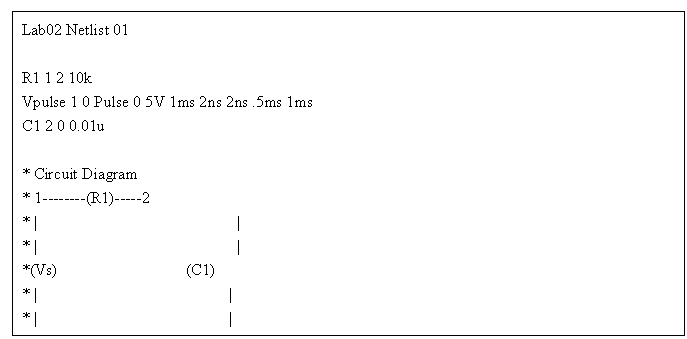Lab 02 Introduction to Pspice

Hung, Bill Chun Wai

Objectives

To get familiar CAD software Spice, in particular, PSpice. To learn the basis of representing circuits.

Procedure/Measurement Report

1.      Perform a transient analysis with PSpice on a RC circuit.

2.      Perform a transient analysis with PSpice on a RLC circuit.

3.      Perform transient analysis with various resistors

4.      Perform a DC analysis with PSpice on a MOSFET circuit.

Results/Analysis

4.1 Lowpass filter CircuitThe result of the circuit is shown in Figure 1. This is a lowpass filter with the coupling capacitor. The coupling capacitor filters out the low frequency because when the frequency is high, the coupling capacitor acts like a voltage resistance voltage source. In other words, the coupling capacitor prevent the output voltage to change too rapidly.

In return, the low frequency, pass the coupling because the capacitor is allowed enough time to charge up or discharge.

4.1 Lowpass filter Circuit with InductorThe H1 indicated in the diagram above is the inductor of 100uH. The inductor is added to the circuit will produce a ringing effect. In other words, the voltage across the resistor oscillates because blow the voltage source side and the inductor-capacitor side is offering voltage to the resistor.

However, due to the small inductance used in the circuit, the oscillation of the circuit is not obvious.

4.2 Lowpass filter with various resistorsThe resistor of value 1 led to an underdamp situation and ringing occurred.

In this part of the experiment, the resistors are replaced by 1 ohm, 50 ohm, 100 ohm, 200 ohm, and 665 ohm. As the resistance increase, the oscillation of the circuit decrease. This is because the resistor acted as a damping force, and when the resistance is larger, the damping force for the oscillation of the circuit is larger. Thus, the oscillation is smaller when the resistance is larger.

4.2 Lowpass filter with various resistorsThe MOSFET circuit shown above is a NMOS with parameters like kp=60e-6, Vto=1, and Lambda=0.05.

This generates a I-V plot of a typical MOSFET circuit indicated in Figure 4.

Conclusions

The lab is to be familiar with PSpice, particularly how to perform transient analysis, DC analysis, and to generate I-V plots. The lab demonstrated the basic techniques to stimulate a circuit and the response of a circuit.